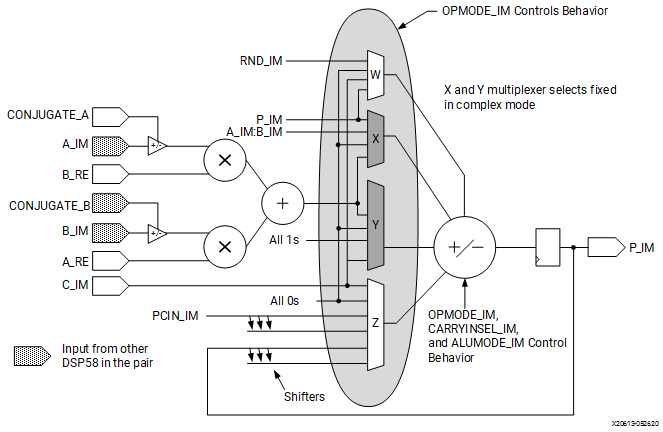# Basic Function and Complex Adder

## Versal ACAP DSP Engine Architecture Manual (AM004)

Document ID
AM004
Release Date
2022-09-11
Revision
1.2.1 English

Two back-to-back DSP58s form one complex arithmetic unit. The two DSP58s together compute,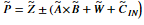where Ã and B̃ are 18-bit complex two’s complement numbers (18 bits real and 18 bits imaginary), W̃, Z̃, and P̃ are complex two’s complement 58-bit fixed-point numbers and C̃in is a one-bit complex carry-in.

The right DSP58 computes (when CONJUGATE_A = CONJUGATE_B = 0),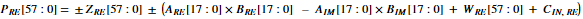while the left DSP58 computes in parallel,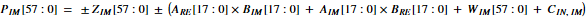As shown in the following figures, the right DSP58 computes the real result PRE and the left DSP58 computes the imaginary result PIM.

Figure 1. Right DSP58 in the Complex Arithmetic Unit to Generate the Real Part of the Result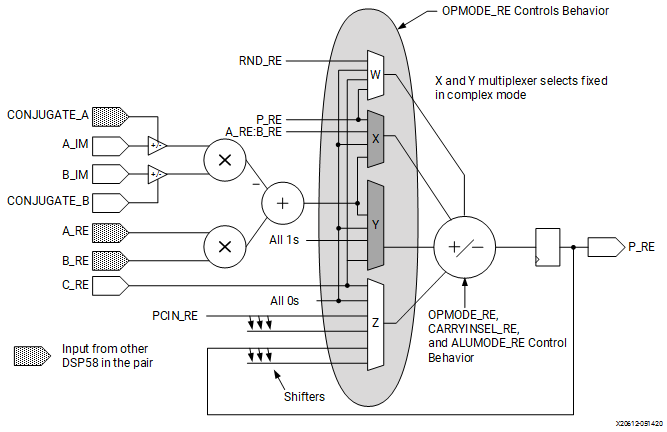Figure 2. Left DSP58 in the Complex Arithmetic Unit to Generate the Imaginary Part of the Result